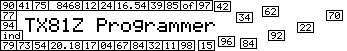## EG ControlsThe envelope is used to produce changes in the sound in relation to the time of the keypresses as you play the keyboard. The EGs are the biggest factor in determining the feel of the sound as you play it.

The EG controls are grouped by operator, with operator 1 on top, followed by operators 2, 3, and 4.

The label for this section is a button which brings up a menu, and this is documented in the section on Menu Buttons.

### AR (Attack Rate)

The attack rate specifies how quickly the operator moves from level zero when you push a key down to its maximum output level as you hold the key. The higher the attack rate, the faster the level increases, as shown on the EG graph.

For example, the sound of a plucked string like a guitar would have a fast attack rate, the sound of a violin would have a medium attack rate, and the sound of a passing car would have a slow attack rate.

### D1R (Decay One Rate)

Once the level reaches the maximum output level, the level begins to decrease at the rate determined by the decay one rate. This rate goes into effect from the maximum level until it reaches the decay one level.

The decay one rate tends to affect the sound of the attack rate. If the decay one rate is fast, it will make the attack sound sharper. For instance, a bell hit with a hard mallet would be emulated with a fast decay one rate, whereas a piano, which doesn't have such a sharp sound in the attack, would be emulated with a slow decay one rate.

If the D1L is set to 15, then the D1R has no effect, since the sound has already "decayed" to 15 as soon as it reaches the attack level, which is fixed at 15.

### D1L (Decay One Level)

The output level decreases from the maximum output level at a rate determined by the decay one rate until it reaches the decay one level. It then begins to decrease at the decay two rate.

The decay one level determines how much bite the attack of the sound has. For a marimba sound, for example, you would give it a fast attack rate and decay one rate, the set the decay one level somewhat low. This will give the impression of a hard mallet hitting the bar.

### D2R (Decay Two Rate)

The decay two rate is the rate at which the output level decreases after reaching the decay one level. It decays at this rate until the level reaches zero. If the D1L is set to zero, then this setting has no effect.

The decay two rate is used for the ringing out of a sound after the character of the attack has played out. For a chime sound, you would use a fairly slow decay two rate, so the sound rings out for awhile as you keep the key pressed.

### RR (Release Rate)

The release rate is the rate at which the output level decreases to zero when the key is released. This can occur at any stage in the envelope.

For example, a typical organ sound turns off immediately when you release the key, so you would use a high release rate. If you wanted to make a chime sound that you could play by quickly hitting the keys as you would hit the chimes, you would use a slow release rate so the sound rings out after you release the key.

### SHFT (EG Shift)

This shifts the zero level of the operator up, so the operator continues to have a certain amount of output when the envelope has fully decayed. The settings are in negative decibels, where the "off" setting is -96dB and is cut in half as the setting is increased: -48dB, -24dB, -12dB. The shift level for operator 1 is permanently set to "off" and can't be changed. This is a limitation of the unit.

This is most useful on modulators for leaving some tone in the sound after the operator has fully decayed to zero. It's pretty much analogous to the L4 level on the DX7.

### EG Graphs

The EG graphs give you an overall impression of how the envelopes affect the sound. For more information see the section on the EG Graphs.